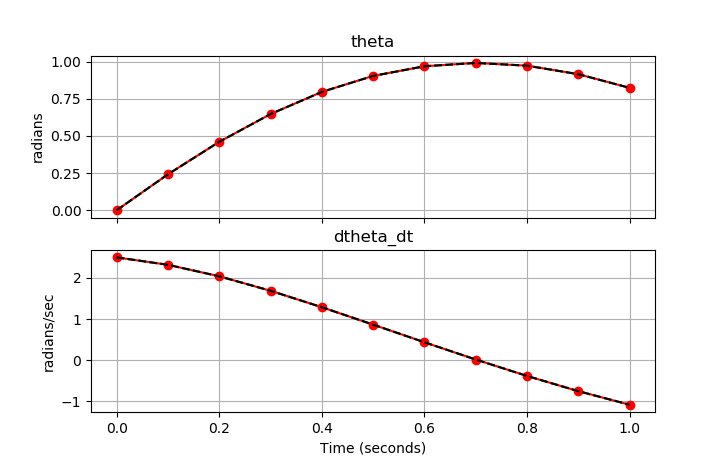## Dynamic Simulation

The DBS file parameter imode is used to control the simulation mode. This option is set to 4 (simultaneous simulation) or 7 (sequential simulation) for dynamic simulation.

 apm.imode = 4 (simultaneous dynamic simulation)
apm.imode = 7 (sequential dynamic simulation)

% MATLAB example
apm_option(server,app,'apm.imode',7);

# Python example
apm_option(server,app,'apm.imode',4)


Like steady-state simulation, dynamic simulation requires a square problem with no degrees of freedom (neqn=nvar). Dynamic simulation has many useful purposes including

• Investigate step response characteristics of a nonlinear model
• Simulate process changes for design, trouble-shooting, or planning
• Perform what-if scenarios
• Simulate a virtual process

Dynamic simulation is the easiest dynamic mode to configure and run. The requirement for a square problem facilitates model convergence as the solver has only to achieve feasibility with the equality constraints.

Example Code (Python GEKKO) with IMODE 4 and 7

Three simulations show simultaneous simulation (IMODE=4), sequential simulation (IMODE=7), and simultaneous simulation in a Python loop (IMODE=4).from gekko import GEKKO
import numpy as np
import matplotlib.pyplot as plt

# Number of timesteps
nt = 11
tm = np.linspace(0, 1, nt)

# Initialize GEKKO
p1 = GEKKO()
p2 = GEKKO()
p3 = GEKKO()

# define model
for p in [p1,p2,p3]:
if p==p3:
p.time = [tm,tm]
else:
p.time = tm

# Model parameters
p.g = p.Const(value=9.81, name='g')
p.l = p.Const(value=2., name='length')
p.m = p.Const(value=1.0, name='mass')
p.f = p.Const(value=0.5, name='friction coefficient')

# State Variables
p.theta = p.Var(value=0, name='angle')
p.dtheta_dt = p.Var(value=2.5, name='angular velocity')

# Equations
p.Equation(p.theta.dt() == p.dtheta_dt)
p.Equation(p.dtheta_dt.dt() == \
-p.g/p.l*p.sin(p.theta) - p.f/p.m*p.dtheta_dt)
p.options.NODES=5

# Solve simultaneously
p1.options.IMODE=4
p1.solve(disp=False)

p2.options.IMODE=7
p2.solve(disp=False)

p3.options.IMODE=4
th = np.ones_like(tm)
dth = np.ones_like(tm)
th = 0
dth = 2.5
import time
for i in range(1,nt):
p3.solve(disp=False)

# record values for plotting
th[i] = p3.theta.value
dth[i] = p3.dtheta_dt.value

# Plot results
fig, axes = plt.subplots(2, 1, sharex=True, figsize=(8,7))

axes.plot(tm, p1.theta.value, 'o-',color='red')
axes.plot(tm, p2.theta.value, ':',color='green')
axes.plot(tm, th, '--',color='black')
axes.set_title("theta")